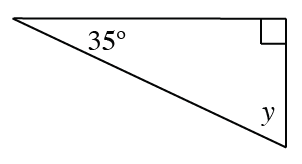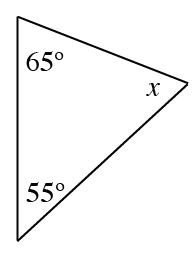### Home > CC1MN > Chapter 10 > Lesson 10.3.2 > Problem10-86

10-86.

Find the measure of the missing angle in each triangle below and then classify the triangle as acute, right, or obtuse.

1.Angle $y$ and the $35º$ angle are complementary.

$y = 55º$

1.The sum of the interior angles in a triangle is always $180º$.